#Function Repository Resource:

# IntegerPartitionQ

Check whether the argument is a weakly decreasing list of positive integers

Contributed by: Wolfram Staff
 ResourceFunction["IntegerPartitionQ"][x] checks whether x is a weakly decreasing list of positive integers.

## Details and Options

An integer partition is a multiset of positive integers and so not ordered. Therefore, any order can be used to represent it. Typically, the order chosen is weakly decreasing, as here; some people choose weakly increasing.

## Examples

### Basic Examples

Here are the 5 integer partitions of 4:

 In:=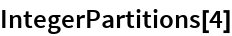Out=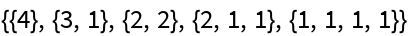They all pass the test for being an integer partition:

 In:=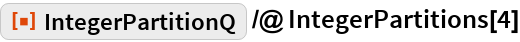Out=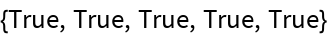The parts all have to be integers:

 In:=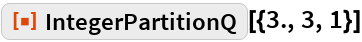Out=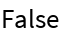The parts have to be positive:

 In:=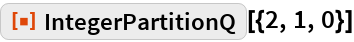Out=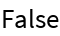The parts have to be in weakly decreasing order:

 In:=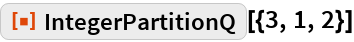Out=## Requirements

Wolfram Language 11.3 (March 2018) or above